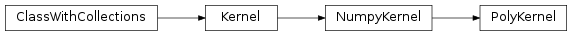# mvpa2.kernels.np.PolyKernel¶class `mvpa2.kernels.np.``PolyKernel`(*args, **kwargs)

Polynomial kernel: K(a,b) = (gamma*a*b.T+coef0)**degree

Attributes

 `descr` Description of the object if any

Methods

 `add_conversion`(typename, methodfull, methodraw) Adds methods to the Kernel class for new conversions `as_ls`(kernel) `as_np`() Converts this kernel to a Numpy-based representation `as_raw_ls`(kernel) `as_raw_np`() Directly return this kernel as a numpy array. `as_raw_sg`(kernel) Converts directly to a Shogun kernel `as_sg`(kernel) Converts this kernel to a Shogun-based representation `cleanup`() Wipe out internal representation `compute`(ds1[, ds2]) Generic computation of any kernel `computed`(\*args, \*\*kwargs) Compute kernel and return self `reset`()

Base Kernel class has no parameters

Attributes

 `descr` Description of the object if any

Methods

 `add_conversion`(typename, methodfull, methodraw) Adds methods to the Kernel class for new conversions `as_ls`(kernel) `as_np`() Converts this kernel to a Numpy-based representation `as_raw_ls`(kernel) `as_raw_np`() Directly return this kernel as a numpy array. `as_raw_sg`(kernel) Converts directly to a Shogun kernel `as_sg`(kernel) Converts this kernel to a Shogun-based representation `cleanup`() Wipe out internal representation `compute`(ds1[, ds2]) Generic computation of any kernel `computed`(\*args, \*\*kwargs) Compute kernel and return self `reset`()